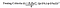# Impurity Measures

Let’s start with what they do and why we need them.

Impurity measures are used in Decision Trees just like squared loss function in linear regression. We try to arrive at as lowest impurity as possible by the algorithm of our choice.

Impurity is presence of more than one class in a subset of data.

So all below mentioned measures differ in formula but align in goal. Watch till the end to know secret highlights of this topic.

Remember this

Make sure you understand that impurity measure is calculated for each leaf node, and its weighted average is the corresponding impurity measure for root node, based on which we say that this feature would become decision feature or not.

Let’s take an example with Entropy and solve to see the exact formulation.

The formula for impurity at leaf node is

After taking weighted average for a feature, we need to check if this feature brings the most reduction in impurity. While using Entropy we do this by Information Gain

where

1. E(Y) should be Entropy before splitting the data over X
2. E(Y|X) is Weighted Entropy after split over X

Example: Consider the Contingency Table asdvv

This should be read as simply Horizontal division is of Liability class labels(Normal and High), while vertical division is that of Credit Rating(Excellent, Good, Poor). So the number 3 in table implies, that out of total 14 companies there were 3 companies which got ‘Excellent rating’ and had ‘Normal Liability’.

Calculation of Entropy for deciding if Credit Rating should be the first split.

First calculate Entropy before splitting

Next entropy over each Leaf node and then weighted average over credit rating split

Note greater the entropy, worse is the current feature for split at present level.

We now calculate information gain(Higher the better, or lower the conditional entropy)

So we get 0.375 as the IG from Credit Rating as the metric for classification of data over liability status. If we had suppose stock price as a independent feature, we would have done the same thing for it as well. Then we would have compared the result for both, and one with higher information gain would have been our first decision variable for splitting.

Now
Impurity Reduction = G(Y) — G(Y|X))

The formula for leaf node is

After weighted average just like above, we calculate

And one offering highest reduction is chosen as decision variable for splitting.

The formula of leaf node is

Often this is a rarely used one.

Another less heard used ones are

The gain ratio “normalizes” the information gain

Impurity measures such as entropy and Gini Index tend to favor attributes that have large number of distinct values. Therefore Gain Ratio is computed which is used to determine the goodness of a split. Every splitting criterion has their own significance and usage according to their characteristic and attributes type.

The Gini Index may encounter problems when the domain of the target attribute is relatively wide. In this case it is possible to employ binary criterion called twoing criteria. This criterion is defined as:Where, p (i/t) denote the fraction of records belonging to class i at a given node t

Little less I could find about it, have a look at this for more understanding.

Highlights:

1. Binary classification: These are primarily used for Binary split, i.e. two leaf nodes, however when multilevel split is there, we can convert them to Binary split like eg. for color(R, G, B) as R or G, B or G, R or B as splitting decision
2. Impurity Index(like Information Gain, Gini Index) are concave functions, and we need to maximize the reduction in impurity. Note as below, graphically also they are Convex Functions.

3. Shapes of the above measures: Continuing from above figure the Impurity Index optimize the choice of feature for splitting but following different paths. Note Classification Error gives a straight line curve as opposed to Entropy or Gini Index.

From this figure, we are trying to compare Entropy(or Gini) method with Classification Error. We are trying to compare Impurity before(Red mark)and after(Green dot) splitting. We want the vertical distance between these two points to maximize, so graphically it is quite intuitive that Classification error being straight Line leaves no space between these two points, however Entropy provides greater space over Gini Index.

4. Difference in use when numerical features instead of categorical: Categorical is easy to follow, while in Numerical our work is just to find average of two corresponding observations(arranged in ascending) and then check the split’s entropy reduction taking each such average as a cutoff. The one providing the max reduction in impurity is chosen as cutoff value.

Problem Solver, Data Science, Actuarial Science, Knowledge Sharer, Hardcore Googler

## More from Shaily jain

Problem Solver, Data Science, Actuarial Science, Knowledge Sharer, Hardcore Googler